# Concrete Calculator – How Much Concrete Do You Need?

Calculate the concrete material needed to pour a slab, patio, footing, column, or post-fill-project in cubic yards or pre-mix bags, and estimate the cost of materials.

Optionally enter the price per cubic yard
\$
*The average price is \$115 - \$150 per cubic yard
Optionally enter the price per cubic yard
\$
*The average price is \$115 - \$150 per cubic yard
Optionally enter the price per cubic yard
\$
*The average price is \$115 - \$150 per cubic yard
Optionally enter the price per cubic yard
\$
*The average price is \$115 - \$150 per cubic yard
Optionally enter the price per cubic yard
\$
*The average price is \$115 - \$150 per cubic yard
Optionally enter the price per cubic yard
\$
*The average price is \$115 - \$150 per cubic yard
Step Dimensions
Optionally specify a custom platform depth for the top step
Optionally enter the price per cubic yard
\$
*The average price is \$115 - \$150 per cubic yard

## Concrete Material Estimate:

### Estimated Cost

\$
Learn how we calculated this below

## How to Calculate Concrete

Concrete, which is a mix of water, aggregate, and cement, is most commonly sold by the cubic yard, but is also available in pre-mixed bags.

It’s important to calculate the amount of material needed as accurately as possible since you often only get one chance for the pour. So, how much concrete will you need?

We’ll cover the process for estimating concrete in detail below, but you can also use the calculator above to simplify the process.

Get Free Project Estimates

Find Qualified Concrete Professionals in Your Area

### Steps to Calculate Cubic Yards of Concrete

• Measure the length, width, and height of the slab, footing, or column.

If the area is complex or multiple pours are needed, break the project into smaller pieces and calculate the concrete needed for each individually.

For instance, when measuring a slab that is not a simple rectangle, try breaking it into different sections and measure the yards needed for each section separately.• Convert dimensions to feet if they aren’t already. Conversion calculators make it easy to convert inches, yards, or meters to feet.
• Multiply the width times the length times the height (W × L × H), or use our volume calculator to find the cubic feet.
• Convert the volume to cubic yards from cubic feet. To do so, divide the cubic footage by 27 to get the yards of concrete needed.
• Round up to the nearest yard as most suppliers will likely not sell fractions of a yard. It is worth asking your supplier if they will sell a partial yard of material, but most do not.
• Estimate how many pre-mixed bags are needed for smaller projects when it isn’t practical to order a truckload of concrete.

The table below shows the coverage of different sizes of pre-mix bags. On average, it will take 90 40lb bags, 60 60lb bags, or 45 80lb bags to fill one cubic yard of concrete.

• Add 5%-10% additional material to account for settling, spillage, and waste. This will ensure you can complete your project in a single pour without needing to order a short load, pay crew overtime, and risk a cold joint that all can occur if you come up short.

### How Much is a Yard of Concrete?

One cubic yard is equal to the volume of a cube with edges that are one yard (three feet) in length.

You might be wondering just how much concrete is in a cubic yard? A cubic yard is equal to 27 cubic feet or 46,656 cubic inches, or 45 80lb bags.

### Concrete Cubic Yards Formulas

Use the following formulas to calculate the concrete needed for various types of pours.

#### Square and Rectangular Areas

The volume for a rectangular space is equal to the length times the width times the height. For slabs, substitute the thickness for the height measurement.

volume = width × length × height

yds3 = width ft × length ft × height ft / 27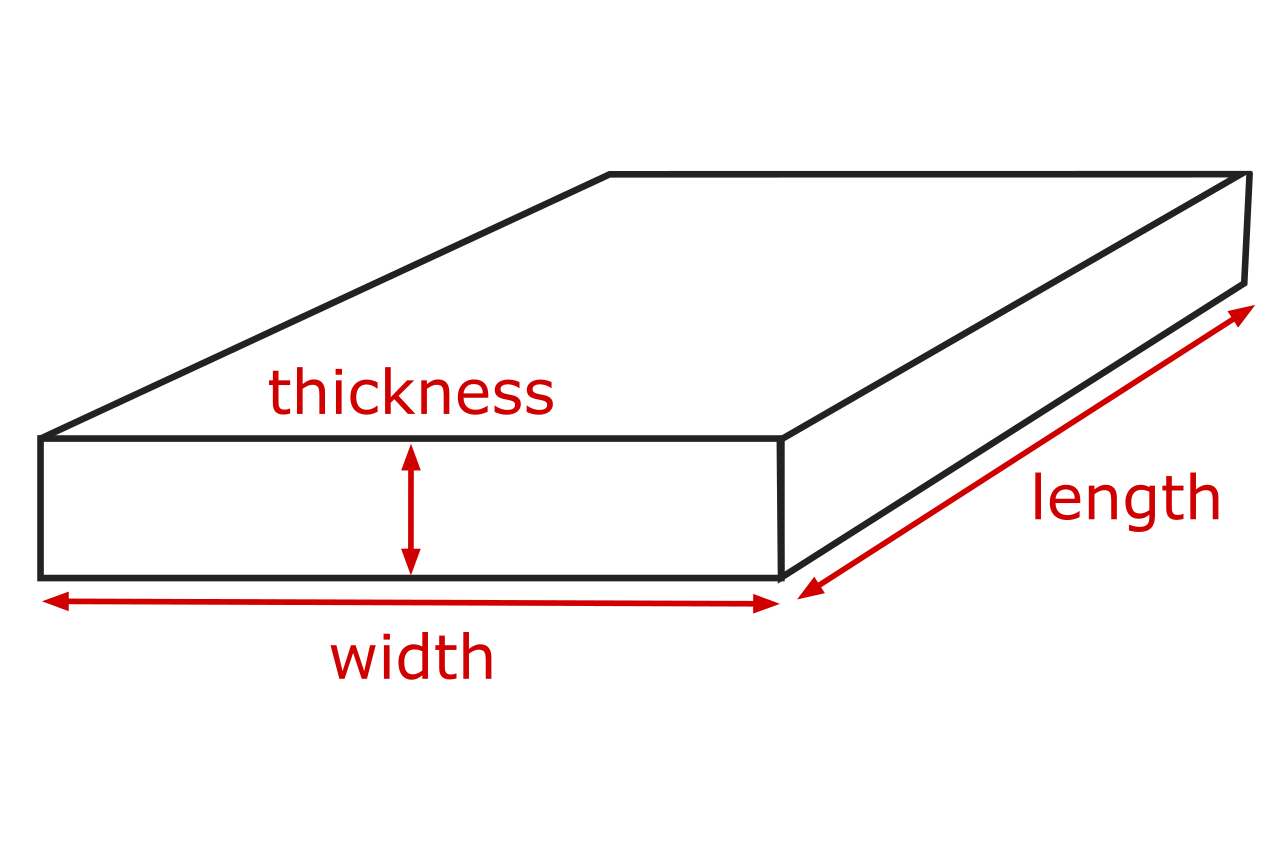#### Circular and Cylindrical Areas

The volume for a circular space is equal to pi times the radius squared times the height. The radius is equal to the diameter divided by two.

volume = π × radius2 × height

yds3 = π × radius ft × radius ft × height ft / 27

π = 3.14159265359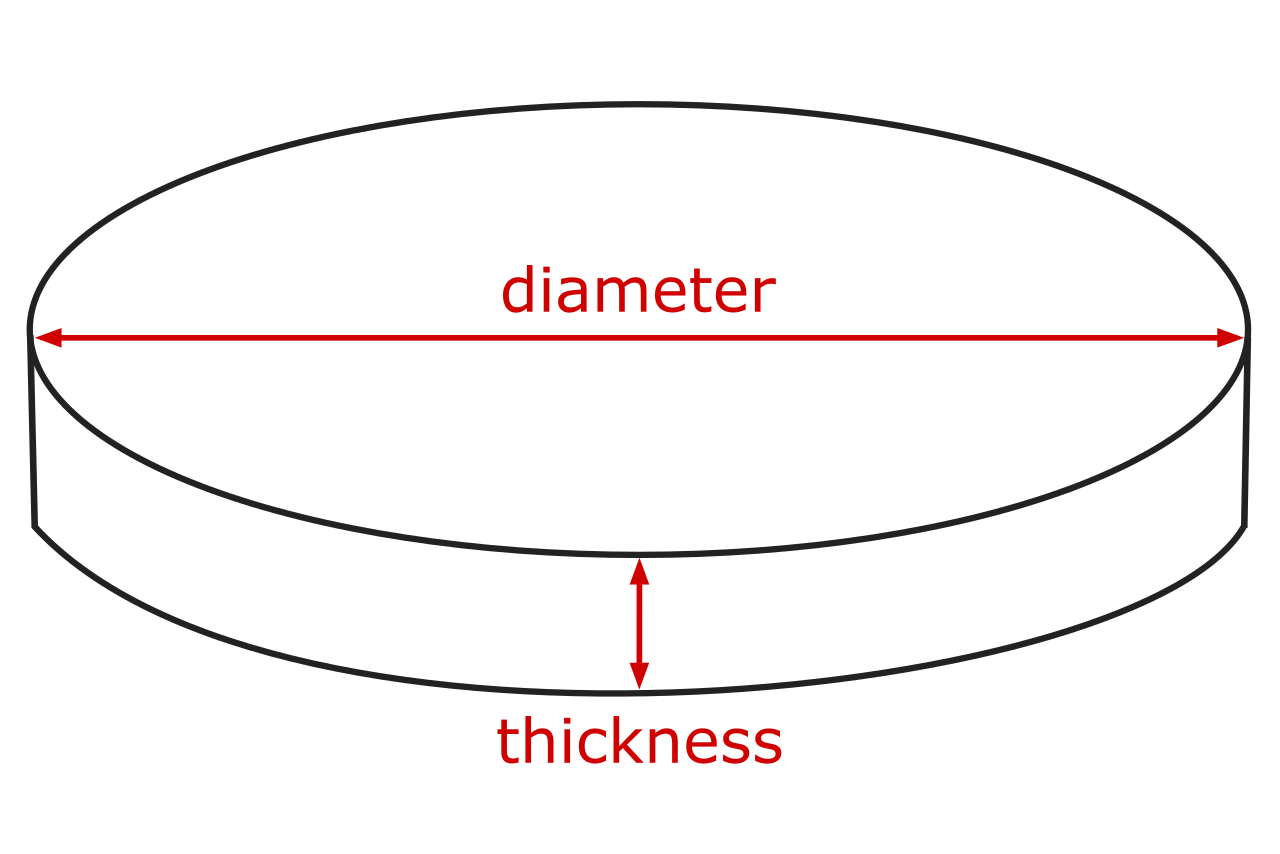#### Steps

Divide the steps into rectangles, then find the volume for each rectangle/cube. Add the volume for each rectangle to find the total volume.

volume = width × length × height
yds3 = width ft × length ft × height ft / 27

Try our concrete steps calculator to easily estimate material for steps and stairs.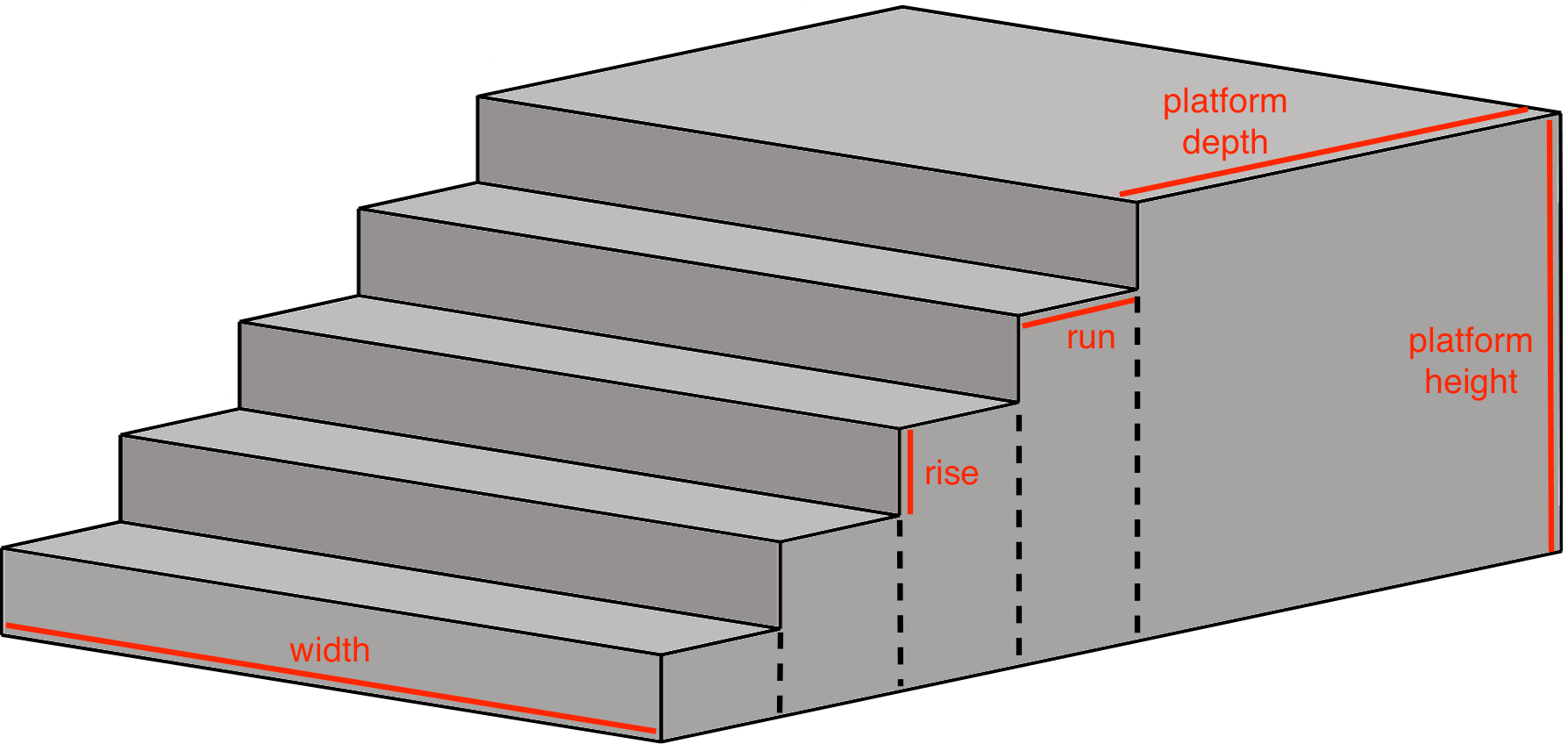For more complex shapes, check out our volume calculator.

## How Many Bags of Concrete do you Need?

So far, we’ve covered how to calculate yards, but if your project is small, you can use pre-mixed bags of concrete. Pre-mixed bags are sold by companies like Quikrete or Sakrete, and come in 40-pound, 60-pound, or 80-pound sizes.

Using bags can be easier than ready-mix when you have many small pours or a small project where a truck is not practical or cost-effective. But, keep in mind that mixing bags on-site can become difficult if you’re pouring a large slab or many footings.

For the most part, ready-mix concrete from a truck is more cost-effective than bags and makes sense if you have a mid-size project and can pour all at once.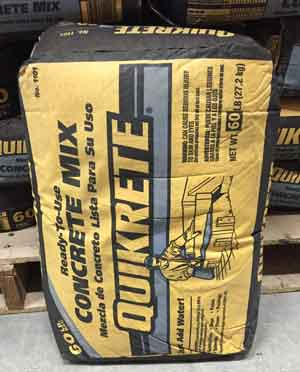So, how many bags of concrete do you need?

Calculating bags is a little less straightforward since each bag size will fill different volumes. Use the calculator above to estimate how many bags you’ll need.

The table below shows the average fill in yards for various pre-mix bag sizes. Check the bag you’re using to find the exact fill-rate for the product.

Table showing the number of pre-bags of concrete needed to fill a volume measured in cubic yards.
Bag Size Pre-mix Bags Needed to Fill
1/4 cu yd 1/2 cu yd 3/4 cu yd 1 cu yd
40 lb bags 23 45 68 90
60 lb bags 15 30 45 60
80 lb bags 12 23 34 45

## How to Calculate Concrete Costs

The cost of a concrete project varies depending on the project’s size and scope, your geographic location, and the type of material used.

### Concrete Cost per Yard

Ready-mix concrete typically starts around \$115 per yard but could cost upwards of \$150 or more, depending on the mix and your location. Consider that there will likely be additional fees for ready-mix for smaller projects, such as delivery or short-load fees.

### Cost per Bag

Pre-mix bags usually range from \$2.50 to \$7, making them a cost-effective choice for small projects.

ready-mix: \$115 – \$150 per cu yd \$2.50 – \$5 per bag \$3 – \$6 per bag \$4.50 – \$7 per bag

The average cost to install a concrete slab is \$6 – \$20 per square foot. Besides the cost of concrete and materials, there are also labor costs for preparing the area, the pour, and finishing.

A standard brush finish will likely be on the low end of the price range, while a decorative finish with coloring will be on the high end of the range. Consider getting a professional concrete installation estimate from contractors in your area for a more accurate cost estimate.

## Tips for Preparing for a Ready-Mix Concrete Pour

Much of the work to pour concrete is done before the truck even arrives at the project. You’ll need to measure and lay out the site and install forms to create the edges of a slab or contain a footing.

Plan a proper base using a gravel or stone calculator to estimate the right amount of base fill.

Use rebar or fiber/steel mesh to reinforce the concrete and to prevent cracking in the future. Use our rebar material and reinforcing mesh calculators to estimate the amount of reinforcement needed for your project.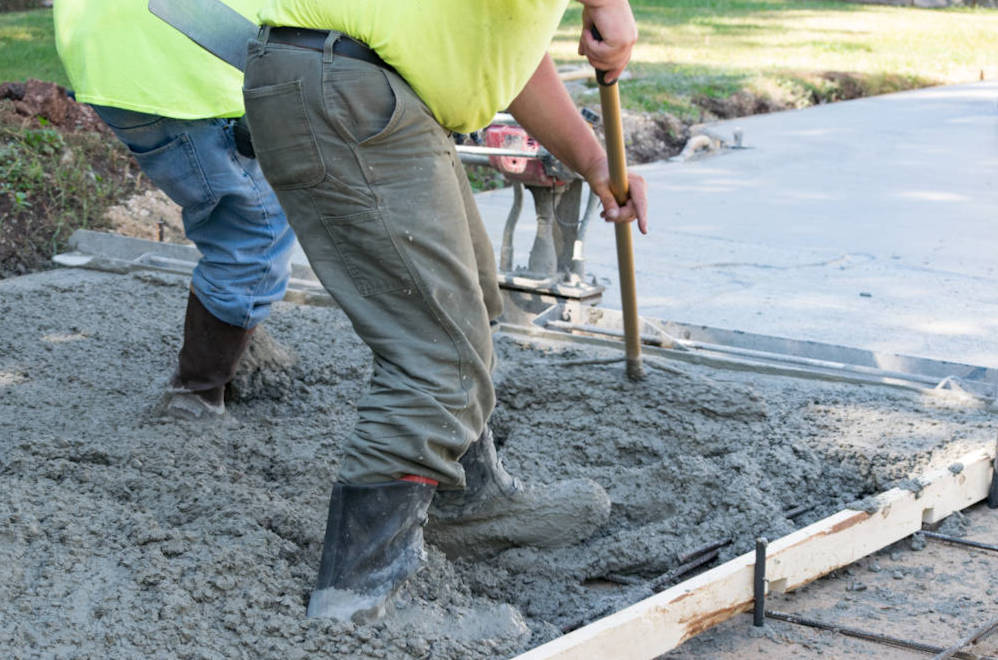Ensure the installation team is ready for the installation and able to begin as soon as the truck arrives to avoid having it set up before the project can be completed and minimize idle time.

If you’re curious how much it weighs, try our concrete weight calculator.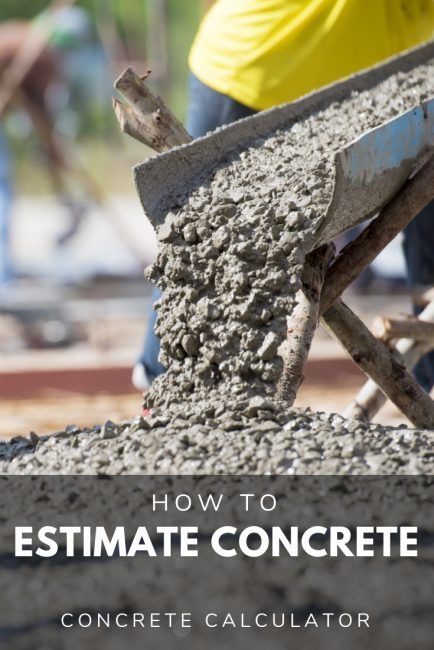## References

1. Family Handyman, How to Estimate a Concrete Order, https://www.familyhandyman.com/article/how-to-estimate-a-concrete-order/